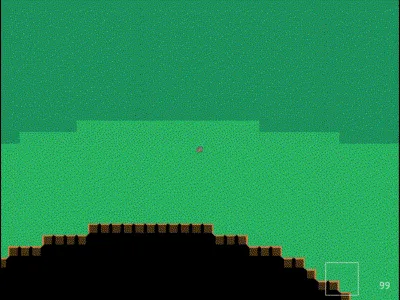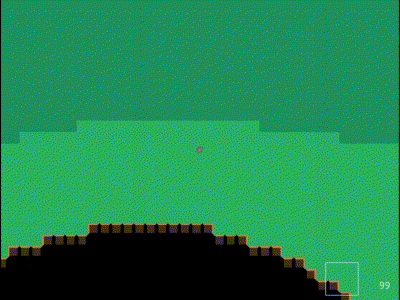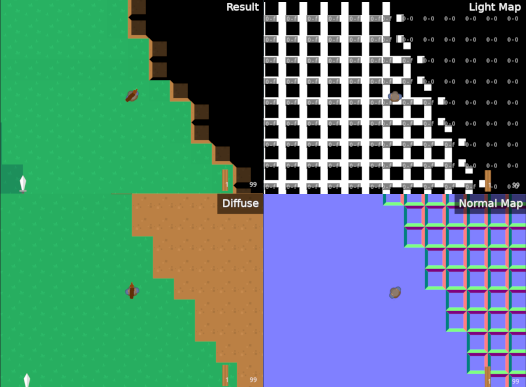# Rendering a topdown world with layers and z-levels using SFML

## Sidebar

Ruben’s Virtual World Project is a game I’ve been working on for almost 4 years now. Recently I rewrote the rendering code to support voxel lighting and multiple z-level - heights of the map.Demonstration of multiple z-layers. The world is rendered as a cross-section, with lower layers shown with a slight fade. This is why you can see below the grass when the players drops down a layer.

## World Layers #

Each z-level has two layers - a tile layer and a floor/terrain layer. Each of these layers has a mesh (VertexArray) which are created in slightly different ways. The floor layer is totally populated, meaning that every position has a quad representing it. The tile layer is sparsely populated, meaning that only positions which have a tile have a matching mesh quad.

Multiple z-levels are rendered one after another, with hidden z-levels not rendered at all.

Lighting is performed by a shader on each mesh, and takes in a three different textures - diffuse, normal, and lightmap. The mesh contains UV co-ordinates which are used to index the diffuse map and normal map, as both are dependent on the type of tile and not the position. The lightmap is indexed using the position.The result and the 3 sources to the lighting shader. The weird breaks on the dirt wall is due to the walls not yet connecting themselves to each other.

Each position has 3x3 pixels in the lightmap, representing the lighting above and from each of the four sides.

A vertex shader is needed to export a relative position to the fragment shader:

``````varying vec4 relativePosition;

void main() {
relativePosition = gl_Vertex;

gl_Position = gl_ModelViewProjectionMatrix * gl_Vertex;
gl_TexCoord = gl_TextureMatrix * gl_MultiTexCoord0;
gl_FrontColor = gl_Color;
}``````

The bulk of the work is done in the fragment shader:

``````uniform sampler2D source; // diffuse map
uniform sampler2D lightmap;
uniform sampler2D normalmap;
uniform float factor; // Lower z-levels from cross-section have higher factors
varying vec4 relativePosition;

// From a normal, calculate how much comes from each direction
vec4 getComponents(vec4 normal) {
vec2 rot = normal.xy*2.f - 1.f;
return vec4(
clamp(-rot.y, 0.f, 1.f),
clamp(rot.x, 0.f, 1.f),
clamp(rot.y, 0.f, 1.f),
clamp(-rot.x, 0.f, 1.f)
);
}

void main() {
// Get light values for each direction
vec2 rel        = floor(relativePosition.xy / 64.f) / 16.f;
vec4 lightAbove = texture2D(lightmap, rel + 0.5f / 16.f);
vec4 lightUp    = texture2D(lightmap, rel + vec2(0.5f, 0.f) / 16.f);
vec4 lightRight = texture2D(lightmap, rel + vec2(0.8f, 0.5f) / 16.f);
vec4 lightDown  = texture2D(lightmap, rel + vec2(0.5f, 0.8f) / 16.f);
vec4 lightLeft  = texture2D(lightmap, rel + vec2(0.f, 0.5f) / 16.f);

// Get normal and weighting for each direction
vec4 normal = texture2D(normalmap, gl_TexCoord.xy);
vec4 rot    = getComponents(normal);

// Leak sides to the above, to make underground wall tops visible
lightAbove = lightAbove + clamp(lightUp + lightRight + lightDown + lightLeft, 0.f, 0.6f)
lightAbove = clamp(lightAbove, 0.f, 1.f);

// Calculate final light level
vec4 lightV = rot*lightUp + rot*lightRight + rot*lightDown + rot*lightLeft + (normal.z*2.f - 1.f)*lightAbove;

// Just support 1D lighting for now
float light = lightV;

// Calculate color
vec4 color = texture2D(source, gl_TexCoord.xy);
if (color < 0.1) {
gl_FragColor = color;
} else {
float u         = 1.0 - clamp(factor, 0.0, 1.0);
const vec4 BLUE = vec4(0, 0.75, 1.0, 1.0);
gl_FragColor    = mix(BLUE, color, u) * u * u * light * light;
gl_FragColor = color;
}
}``````

This probably isn’t the best way to do it. This is one of the first shaders I’ve ever written, and graphics isn’t my thing.